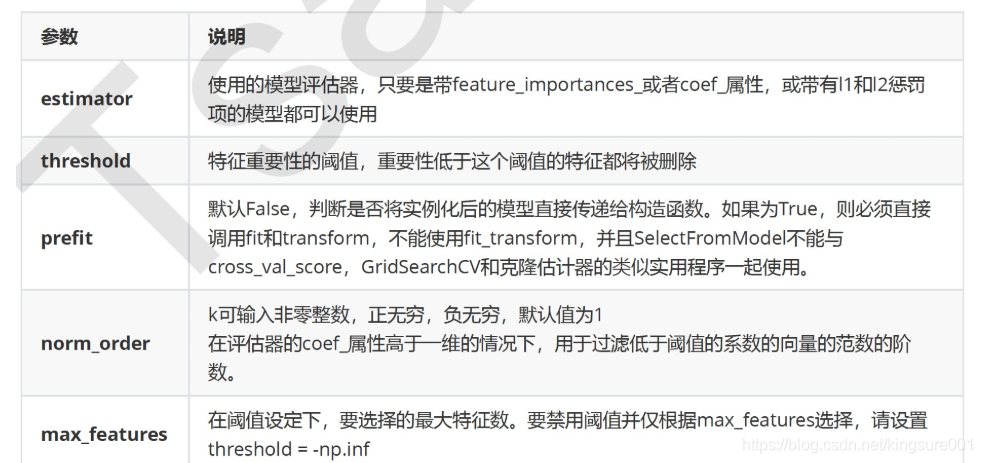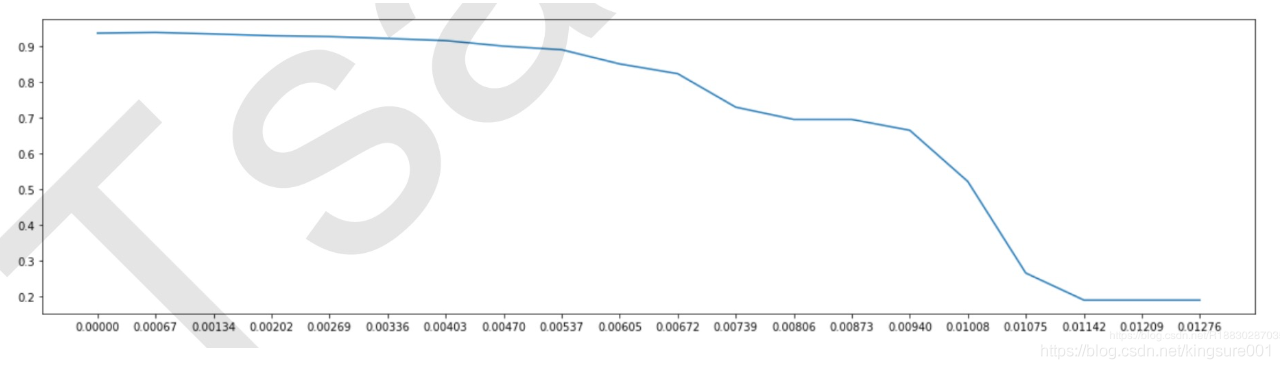﻿• 价格透明
• 信息保密
• 进度掌控
• 售后无忧# 嵌入法：

## 2、嵌入法挑选特征模型feature_selection.SelectFromModel

``class sklearn.feature_selection.SelectFromModel (estimator, threshold=None, prefit=False, norm_order=1,max_features=None)````````from sklearn.feature_selection import SelectFromModel
from sklearn.ensemble import RandomForestClassifier as RFC
RFC_ = RFC(n_estimators =10,random_state=0) #先随机森林的实例化
X_embedded = SelectFromModel(RFC_,threshold=0.005).fit_transform(X,y)#
#在这里我只想取出来有限的特征。0.005这个阈值对于有780个特征的数据来说，是非常高的阈值
#因为平均每个特征只能够分到大约0.001的feature_importances_
X_embedded.shape #（42000，47）
#模型的维度明显被降低了

#同样的，我们也可以画学习曲线来找最佳阈值
#======【TIME WARNING：10 mins】======#
import numpy as np
import matplotlib.pyplot as plt
threshold = np.linspace(0,(RFC_.fit(X,y).feature_importances_).max(),20)
#0到feature_importances_最大值平均取20个
score = []
for i in threshold:
X_embedded = SelectFromModel(RFC_,threshold=i).fit_transform(X,y)
once = cross_val_score(RFC_,X_embedded,y,cv=5).mean()
score.append(once)
plt.plot(threshold,score)
plt.show()
``````挑选一个threshold=0.00067来验证一下模型的效果，特征个数瞬间缩小到324多，这比我们在方差过滤的时候选择中位数过滤出来的结果392列要小，并且交叉验证分数0.9399高于方差过滤后的结果0.9388，这是由于嵌入法比方差过滤更具体到模型的表现的缘故，换一个算法，使用同样的阈值，效果可能就没有这么好了。### 低价透明### 金牌服务### 信息保密